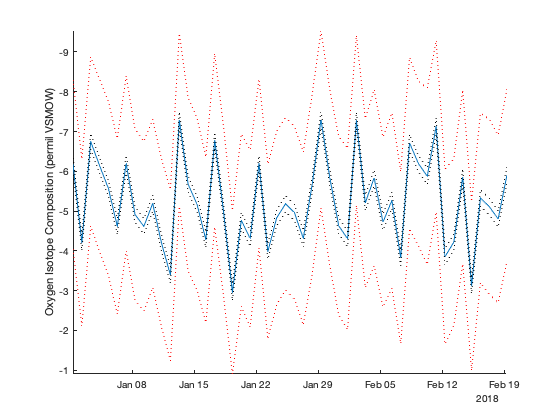# ts_normstrap documentation

ts_normstrap performs a bootstrap uncertainty analysis on a time series given an uncertainty value at each step assuming a normal probability distribution. Bootstrapping means estimating a value at each time point within particular uncertainty bounds with a given probability distribution. Ultimately, the goal is to generate several realizations of the time series and provide confidence intervals at each time step.

Back to Climate Data Tools Contents

## Syntax

```tsb = ts_normstrap(ts)
tsb = ts_normstrap(ts,e)
tsb = ts_normstrap(ts,E)
tsb = ts_normstrap(ts,'nboot',nboot)
[tsb,Nts] = ts_normstrap(...)```

## Description

tsb = ts_normstrap(ts) calculates confidence intervals for a given timeseries after randomly subsampling vector ts 1000 times at each point with a normal probability, assuming an uncertainty of 1 standard deviation of the overall time series ts. The output tbs is a length(ts) x2 matrix containg the +/- 1 standard deviation bounds of time series ts. Note that ts is a vector without time dimensions as the bounds are returned at the points of query.

tsb = ts_normstrap(ts,e) specifies the uncertainty value e from which the uncertainty distribution at each step in the vector ts is calculated and thereby overrides the default value of 1 standard deviation of ts.

tsb = ts_normstrap(ts,E) specifies a vector E containing uncertainty values at each step from which the uncertainty distribution in vector ts is calculated.

tsb = ts_normstrap(...,'nboot',nboot) specifies the number of bootstrap samples. Default is 1000, meaning 1000 random time series are calculated.

[tsb,Nts] = ts_normstrap(...) also returns the 1000 (or the specified number of) randomly generated time series with given uncertainty.

[tsb,Nts] = ts_normstrap(...) also returns the 1000 (or the specified number of) randomly generated time series subsampled with the specified uncertainty.

## Example

This example performs a bootstrap analysis of a randomly generated time series of 50 points, which we shall assume to be 50 measurements of oxygen isotopes in water with a mean value around -5 permil VSMOW.

```iso_ts = -5 + randn(50,1);

% Let's say they were continuously sampled over 50 days in 2018
t1 = datetime(2018,1,1,8,0,0);
t = t1:t1+49;
```

Let's plot the data

```figure
plot(t,iso_ts)
box off
axis tight
ylabel 'Oxygen Isotope Composition (permil VSMOW)'
set(gca,'ydir','reverse') % flips the direction of the Y-Axis
```Now we want uncertainty bounds for the time series

```tsb = ts_normstrap(iso_ts);

% This will, by default, give uncertainty bounds close the the standard
% deviation of the overall |iso_ts| timeseries
overall_sd = std(iso_ts)
default_bootstrap_uncertainty = mean(tsb)

% Let's plot it up on the original plot as a 2-sigma bound (multiply by 2)
hold on;
plot(t,iso_ts+2.*tsb,':r')
plot(t,iso_ts-2.*tsb,':r')
```
```overall_sd =
1.0830
default_bootstrap_uncertainty =
1.0817
```Now let's be a little more specific and specify the analytical uncertainty of the oxygen isotopic measurement as 0.1 permil

```tsb = ts_normstrap(iso_ts,0.1);

specified_bootstrap_uncertainty = mean(tsb)
```
```specified_bootstrap_uncertainty =
0.1002
```

Let's plot the new, better uncertainty, also as a 2-sigma bound

```hold on;
plot(t,iso_ts+2.*tsb,':k')
plot(t,iso_ts-2.*tsb,':k')
```Now let's compare a low number of bootstrap samples to a high number of samples

```tsb_low = ts_normstrap(iso_ts,0.1,'nboot',3);
tsb_high = ts_normstrap(iso_ts,0.1,'nboot',500);

low_bootstrap_uncertainty = mean(tsb_low)
high_bootstrap_uncertainty = mean(tsb_high)
```
```low_bootstrap_uncertainty =
0.0956
high_bootstrap_uncertainty =
0.0997
```

Let's plot them both now:

```figure; hold on;
plot(t,iso_ts)
box off
axis tight
ylabel 'Oxygen Isotope Composition (permil VSMOW)'
set(gca,'ydir','reverse') % flips the direction of the Y-Axis

plot(t,iso_ts+2.*tsb_low,'--r')
plot(t,iso_ts-2.*tsb_low,'--r')

plot(t,iso_ts+2.*tsb_high,'--g')
plot(t,iso_ts-2.*tsb_high,'--g')
```Notice that sometimes the low number indicates higher uncertainty and sometimes it shows a lower uncertainty. Keep in mind that larger number of bootstrap samples will generally converge to some value.

For fun, let's plot all the time series that we have generated!

```[tsb,Nts] = ts_normstrap(iso_ts,0.08,'nboot',500);

figure;hold on;
plot(t,Nts,'color',[0.7 0.7 0.7]) % In gray
plot(t,iso_ts,'ko-','linewidth',1.5); % In black
```## References

The original bootstrap was introduced formally to the literature by Eforon in 1979: Efron, B., 1979: Bootstrap methods: another look at the jackknife. Ann. Stat. 7, 1-26. doi:10.1007/978-1-4612-4380-9_41.

Although this is a rather dense read! Here are some (paleoclimate) applications:

Thirumalai, K., T. M. Quinn, and G. Marino, 2016: Constraining past seawater delta-18-O and temperature records developed from foraminiferal geochemistry, Paleoceanography doi:10.1002/2016PA002970.

Carré, M., J. P. Sachs, J. M. Wallace, and C. Favier, 2012: Exploring errors in paleoclimate proxy reconstructions using Monte Carlo simulations: paleotemperature from mollusk and coral geochemistry, Clim. Past, 8(2), 433-450. doi:10.5194/cp-8-433-2012.

The first figure in both of these papers provide a flowchart to understand basic schematics of the bootstrap in age uncertainty.

## Author Info

The ts_normstrap function and supporting information were written for The Climate Data Toolbox for Matlab by Kaustubh Thirumalai of the University of Arizona, January 2019.